# SSAT Middle Level Math : How to find the decimal equivalent of a fraction

## Example Questions

1 2 4 Next →

### Example Question #128 : Understand Decimal Notation For Fractions, And Compare Decimal Fractions

What decimal is equivalent to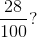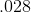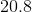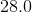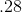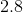Explanation:is twenty-eight hundredths.is twenty-eight hundredths. When we say a decimal, we say the number and add the place-value of the last digit.

### Example Question #129 : Understand Decimal Notation For Fractions, And Compare Decimal Fractions

What decimal is equivalent to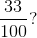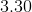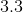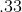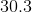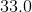Explanation: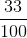is thirty-three hundredths.is thirty-three hundredths. When we say a decimal, we say the number and add the place-value of the last digit.

### Example Question #121 : Understand Decimal Notation For Fractions, And Compare Decimal Fractions

What decimal is equivalent to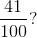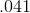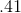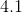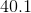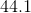Explanation: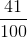is forty-one hundredths.is forty-one hundredths. When we say a decimal, we say the number and add the place-value of the last digit.

### Example Question #851 : Fractions

What is the decimal equivalent to the following fraction?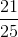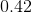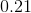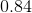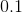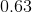Explanation:

When solving for a decimal from a fraction, you have two options. You can either do long division, and divide it out. How many times does 25 go into 21? Or the second option is setting the denominator as 100.

In this case, 25 goes into 100 evenly.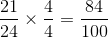.

If the denominator is 100, then the numerator is the number after the decimal point.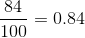.

### Example Question #851 : Fractions

Write 0.74 as a fraction in lowest terms.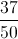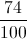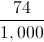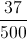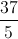Explanation:

This decimal has its last nonzero digit in the hundredths place; this number is equal to "seventy-four one-hundredths". As a fraction, this is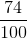This is not in lowest terms, since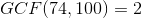.

Reduce: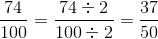1 2 4 Next →